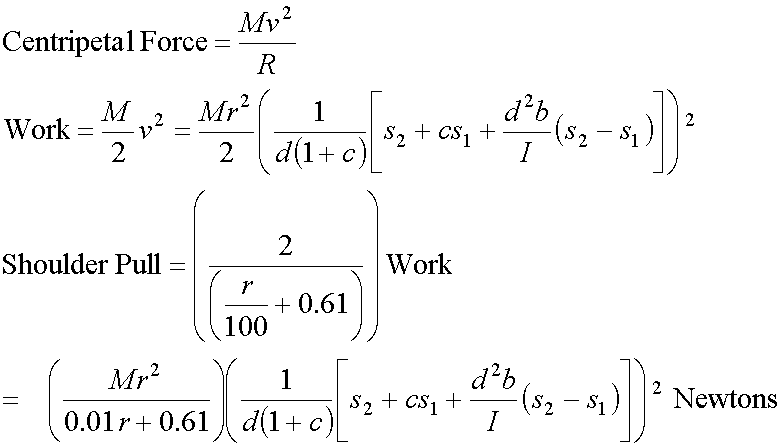## Derivation of the Shoulder Pull Formula

Shoulder Pull is the centripetal force, in Newtons,with the shoulder being a distance R (= 0.61 + r/100) meters from the racquet mass center. The Shoulder Pull force, measured in units of Newtons (1 Newton = 0.225 lb.) attributable to the racquet alone is derived from the Work formula and the general formula for centripetal force (centrifugal force). See the accompanying remarks. A complete description of all variables used in this derivation follows at the end.I b = moment of inertia (swing weight) of racquet, in kilograms·centimeters² = mass of ball, in kilograms = distance from axis of rotation to impact point, in centimeters = ball velocity, in meters-per-second (positive is away from player) = distance from axis of rotation to mass center, in centimeters = linear velocity of mass center, in meters-per-second = mass of racquet, in kilograms = coefficient of restitution of the racquet/ball system